Open in App
Not now

# SQL general functions | NVL, NVL2, DECODE, COALESCE, NULLIF, LNNVL and NANVL

• Difficulty Level : Easy
• Last Updated : 18 Dec, 2019

In this article, we’ll be discussing some powerful SQL general functions, which are – NVL, NVL2, DECODE, COALESCE, NULLIF, LNNVL and NANVL.

These functions work with any data type and pertain to the use of null values in the expression list. These are all single row function i.e. provide one result per row.

• NVL(expr1, expr2) : In SQL, NVL() converts a null value to an actual value. Data types that can be used are date, character and number. Data type must match with each other i.e. expr1 and expr2 must of same data type.
Syntax –

```NVL (expr1, expr2)
```

expr1 is the source value or expression that may contain a null.
expr2 is the target value for converting the null.

Example –

```SELECT  salary, NVL(commission_pct, 0),
(salary*12) + (salary*12*NVL(commission_pct, 0))
annual_salary FROM employees;
```

Output :• NVL2(expr1, expr2, expr3) : The NVL2 function examines the first expression. If the first expression is not null, then the NVL2 function returns the second expression. If the first expression is null, then the third expression is returned i.e. If expr1 is not null, NVL2 returns expr2. If expr1 is null, NVL2 returns expr3. The argument expr1 can have any data type.

Syntax –

```NVL2 (expr1, expr2, expr3)
```

expr1 is the source value or expression that may contain null
expr2 is the value returned if expr1 is not null
expr3 is the value returned if expr1 is null

Example –

```SELECT last_name, salary, commission_pct,
NVL2(commission_pct, ’SAL+COMM’, ’SAL’)
income FROM employees;
```

Output :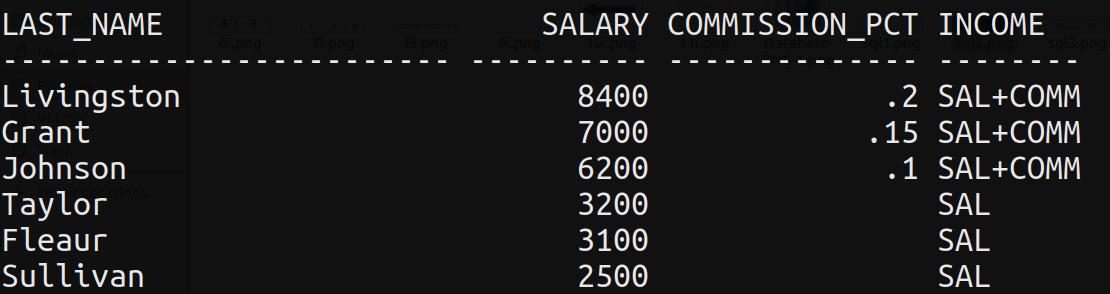• DECODE() : Facilitates conditional inquiries by doing the work of a CASE or IF-THEN-ELSE statement.
The DECODE function decodes an expression in a way similar to the IF-THEN-ELSE logic used in various languages. The DECODE function decodes expression after comparing it to each search value. If the expression is the same as search, result is returned.
If the default value is omitted, a null value is returned where a search value does not match any of the result values.

Syntax –

```DECODE(col|expression, search1, result1
[, search2, result2,...,][, default])
```

Example –

```SELECT last_name, job_id, salary,
DECODE(job_id, ’IT_PROG’, 1.10*salary,
’ST_CLERK’, 1.15*salary,
’SA_REP’, 1.20*salary,salary)
REVISED_SALARY FROM employees;
```

Output :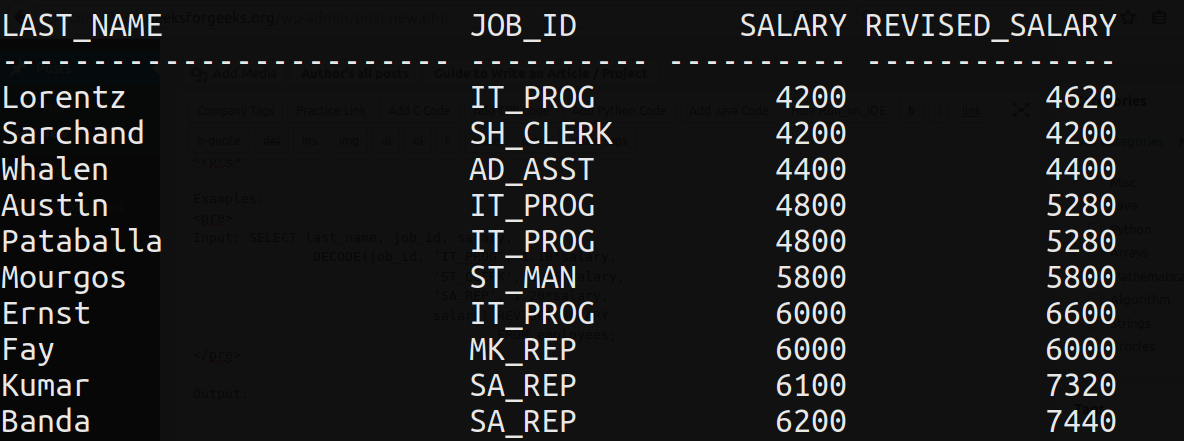• COALESCE() : The COALESCE() function examines the first expression, if the first expression is not null, it returns that expression; Otherwise, it does a COALESCE of the remaining expressions.
The advantage of the COALESCE() function over the NVL() function is that the COALESCE function can take multiple alternate values. In simple words COALESCE() function returns the first non-null expression in the list.

Syntax –

```COALESCE (expr_1, expr_2, ... expr_n)
```

Examples –

```SELECT last_name,
COALESCE(commission_pct, salary, 10) comm
FROM employees ORDER BY commission_pct;
```

Output :• NULLIF() : The NULLIF function compares two expressions. If they are equal, the function returns null. If they are not equal, the function returns the first expression. You cannot specify the literal NULL for first expression.

Syntax –

```NULLIF (expr_1, expr_2)
```

Examples –

```SELECT LENGTH(first_name) "expr1",
LENGTH(last_name) "expr2",
NULLIF(LENGTH(first_name),LENGTH(last_name))
result FROM employees;
```

Output :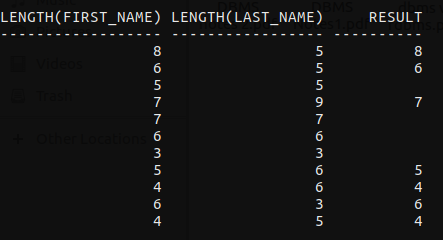• LNNVL() : LNNVL evaluate a condition when one or both operands of the condition may be null. The function can be used only in the WHERE clause of a query. It takes as an argument a condition and returns TRUE if the condition is FALSE or UNKNOWN and FALSE if the condition is TRUE.

Syntax –

```LNNVL( condition(s) )
```

Examples –

```SELECT COUNT(*) FROM employees
WHERE commission_pct < .2;
```

Output :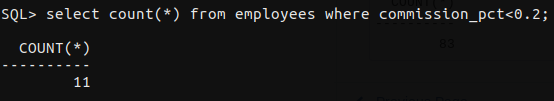• Now the above examples does not considered those employees who have no commission at all.
To include them as well we use LNNVL()

```SELECT COUNT(*) FROM employees
WHERE LNNVL(commission_pct >= .2);
```

Output :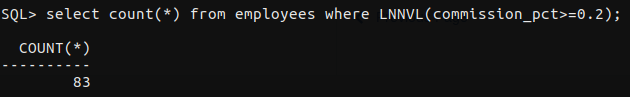• NANVL() : The NANVL function is useful only for floating-point numbers of type BINARY_FLOAT or BINARY_DOUBLE. It instructs the Database to return an alternative value n2 if the input value n1 is NaN (not a number). If n1 is not NaN, then database returns n1. This function is useful for mapping NaN values to NULL.

Syntax –

`NANVL( n1 , n2 )`

Consider the following table named nanvl_demo :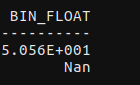Example –

```SELECT bin_float, NANVL(bin_float,0)
FROM nanvl_demo;
```

Output :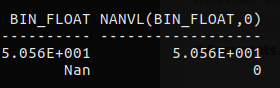Reference: Introduction to Oracle9i SQL(Volume-1 Book)

My Personal Notes arrow_drop_up